# zticks

Set or query z-axis tick values

## Syntax

``zticks(ticks)``
``zt = zticks``
``zticks('auto')``
``zticks('manual')``
``m = zticks('mode')``
``___ = zticks(ax,___)``

## Description

example

````zticks(ticks)` sets the z-axis tick values, which are the locations along the z-axis where the tick marks appear. Specify `ticks` as a vector of increasing values; for example, `[0 2 4 6]`. This command affects the current axes.```
````zt = zticks` returns the current z-axis tick values as a vector.```

example

````zticks('auto')` sets an automatic mode, enabling the axes to determine the z-axis tick values. Use this option if you change the tick values and then want to set them back to the default values. ```
````zticks('manual')` sets a manual mode, freezing the z-axis tick values at the current values. Use this option if you want to retain the current tick values when resizing the axes or adding new data to the axes.```
````m = zticks('mode')` returns the current z-axis tick values mode, which is either `'auto'` or `'manual'`. By default, the mode is automatic unless you specify tick values or change the mode to manual.```

example

````___ = zticks(ax,___)` uses the axes specified by `ax` instead of the current axes. Specify `ax` as the first input argument for any of the previous syntaxes.```

## Examples

collapse all

Create a surface plot. Display tick marks along the z-axis at the values -8, 0, and 8. Then, specify a label for each tick mark.

```[x,y,z] = peaks; surf(x,y,z) zticks([-8 0 8]) zticklabels({'z = -8','z = 0','z = 8'})```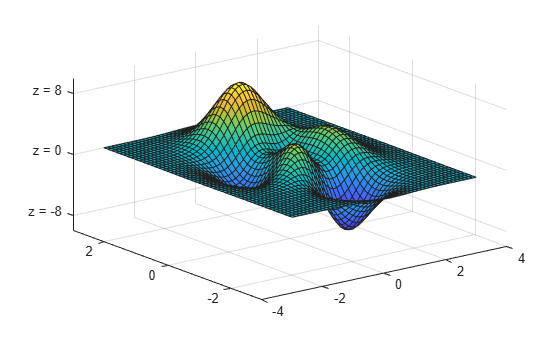Display tick marks along the z-axis at nonuniform values between -10 and 10. MATLAB® labels the tick marks with the numeric values.

```[x,y,z] = peaks; surf(peaks) zticks([-10 -2.5 0 2.5 10])```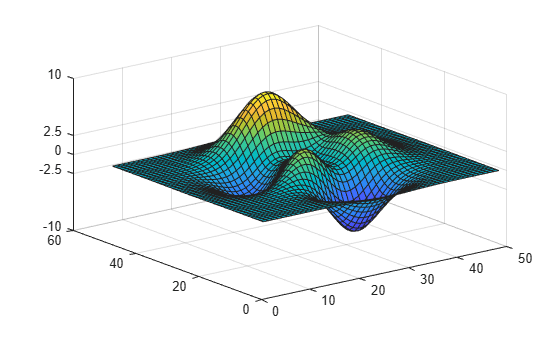Display tick marks along the z-axis at increments of 2, starting from -10 and ending at 10.

```[x,y,z] = peaks; surf(peaks) zticks([-10:2:10])```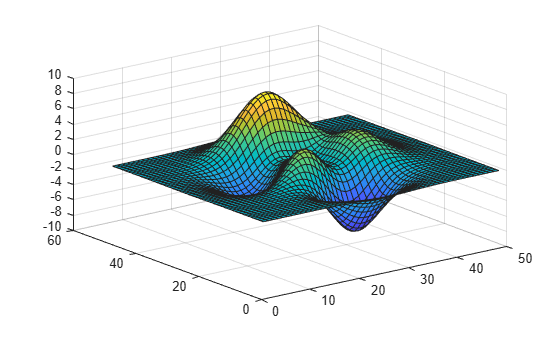Create a plot and specify the z-axis tick values. Then, set the z-axis tick values back to the default values.

```t = 0:pi/50:10*pi; st = sin(t); ct = cos(t); plot3(st,ct,t) zticks(0:8:40)```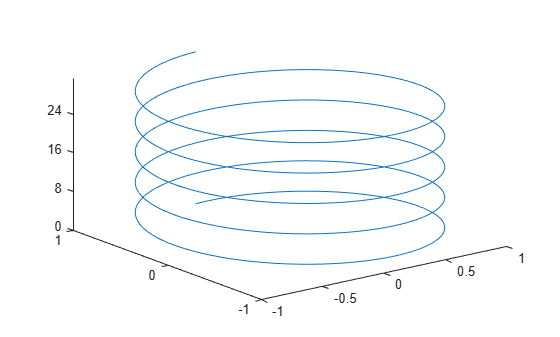`zticks('auto')`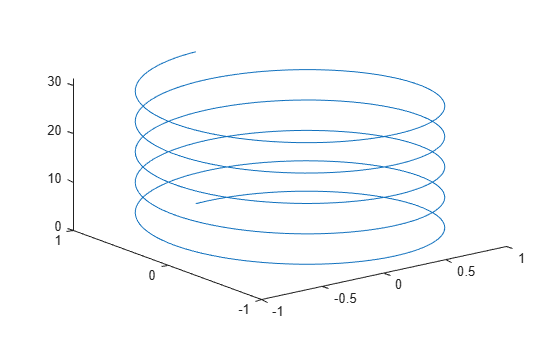Starting in R2019b, you can display a tiling of plots using the `tiledlayout` and `nexttile` functions. Call the `tiledlayout` function to create a 2-by-1 tiled chart layout. Call the `nexttile` function to create the axes objects `ax1` and `ax2`. Create two 3-D stem plots, and set the z-axis tick values for the upper plot by passing `ax1` as the first input argument to the `zticks` function.

```tiledlayout(2,1) ax1 = nexttile; stem3(ax1,4*rand(5)) zticks(ax1,[0 1.5 3.25]) ax2 = nexttile; stem3(ax2,4*rand(5))```Remove the tick marks along the z-axis by specifying the tick values as an empty array. Removing the tick marks also removes any grid lines in the z direction.

```[x,y,z] = peaks; mesh(x,y,z) zticks([])```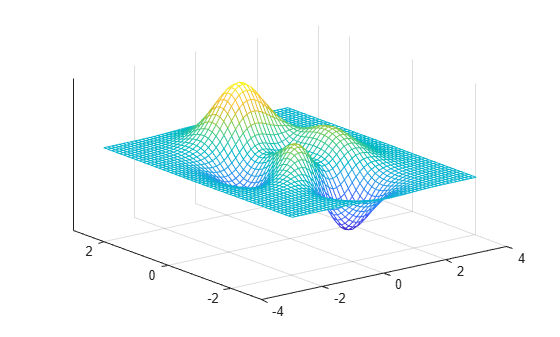## Input Arguments

collapse all

Tick values, specified as a vector of increasing values. If you do not want tick marks along the z-axis, specify an empty vector `[]`.

You can specify the tick values as numeric, categorical, datetime, or duration values. However, the type of values that you specify must match the type of values along the z-axis.

Example: `zticks([pi 2*pi 3*pi 4*pi])`

Example: `zticks(0:10:100)`

Example: `zticks([])`

Note

To specify the tick labels, use the `zticklabels` function.

Data Types: `single` | `double` | `int8` | `int16` | `int32` | `int64` | `uint8` | `uint16` | `uint32` | `uint64` | `categorical` | `datetime` | `duration`

Target axes, specified as an `Axes` object or an array of `Axes` objects.

If you do not specify this argument, then `zticks` modifies the current axes.

## Output Arguments

collapse all

Current tick values, returned as a vector.

Current mode, returned as one of these values:

• `'auto'` — Automatically determine the z-axis tick values.

• `'manual'` — Use manually specified z-axis tick values.

collapse all

### Tick Values

The tick values are the locations along the z-axis where the tick marks appear. The tick labels are the labels that you see next to each tick mark. Set the tick values using the `zticks` function. Set the corresponding tick labels using the `zticklabels` function.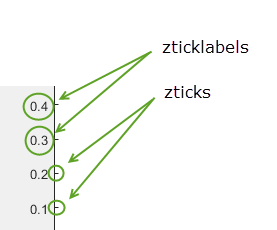## Algorithms

The `zticks` function sets and queries several axes properties related to the z-axis tick values.

• `ZTick` — Property that stores the z-axis tick values.

• `ZTickMode` — Property that stores the z-axis tick value mode. When you set the z-axis tick values, this property changes to `'manual'`.

## Version History

Introduced in R2016b